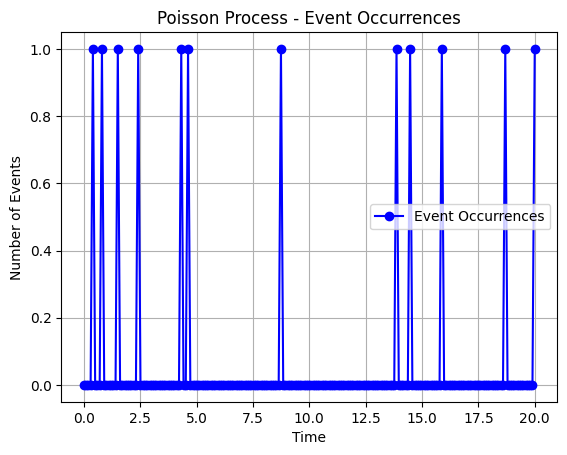Course Content

# Probability Theory Basics

Probability Theory Basics

##Poisson Distribution

Assume that we have some sequence of events that occurred in some period of time with the following properties:

1. Events are independent.
2. The simultaneous occurrence of two or more events hasмlow probability (in this case, simultaneity is implied in the context of the occurrence of events in an extremely small time interval - up to seconds).
3. Probabilistic characteristics of the occurrence of an event do not depend on time.

In this case, this set of events is called Poisson point process. The examples of Poisson point processes are:

• the arrival of cosmic particles on the counter;
• client requests to the server on a certain day of the week;
• road accidents on a certain section of the road on a certain day;
• insurance cases with clients of a certain insurance company.Note

It is important to understand the difference between the Bernoulli and Poisson processes. In the case of the Bernoulli process, we independently conduct an experiment and count the number of successes. At the same time, the Poisson process describes events in nature that we do not directly influence but only observe their appearance.

The Poisson distribution is a discrete probability distribution representing the number of events occurring in a fixed time interval in the Poisson point process. This distribution has one parameter representing the average number of events occurring in a one-time unit.
Let's solve the following task using Poisson distribution:

In a call center, calls are received at an average rate of `5` calls per minute. What is the probability of receiving from `290` to `310` calls in an hour?In the code above, we used `.pmf()` method of `scipy.stats.poisson` class to calculate probability in each of. the points `290`, `291`, ... , `310` and summarized al these probabilities to calculate final result.
Parameter `mu` determines the average number of accidents over a period of time.
If you want to calculate the probability for a different period of time, then in the `mu` parameter, specify the average number of events in the desired period.